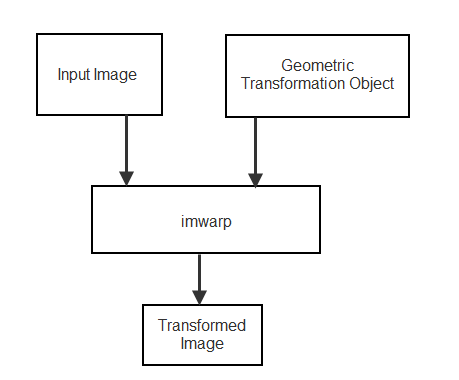## 2-D and 3-D Geometric Transformation Process Overview

To perform a 2-D or 3-D geometric transformation, first create a geometric transformation object that stores information about the transformation. Then, pass the image to be transformed and the geometric transformation object to the `imwarp` function. You optionally can provide spatial referencing information about the input image to `imwarp`.### Create Geometric Transformation Object

Different types of geometric transformation objects store different information about the transformation.

There are several ways to create a geometric transformation object.

Approach to Create Transformation Object`affine2d``affine3d`

`rigid2d`

`rigid3d`

`projective2d`

`geometricTransform2d`

`geometricTransform3d`

Other Transformation Objects
Define Transformation MatrixXXXX
Define Custom Point-Wise Mapping Function    X
Estimate Transformation from Control Point PairsX  X X
Estimate Transformation Using Similarity OptimizationXX
Estimate Transformation Using Phase CorrelationX

#### Define Transformation Matrix

If you know the transformation matrix for the geometric transformation you want to perform, then you can create a `rigid2d`, `affine2d`, `projective2d`, `rigid3d`, or `affine3d` geometric transformation object directly. For more information about creating a transformation matrix, see Matrix Representation of Geometric Transformations.

The following example defines the transformation matrix for a 2-D translation and creates an `affine2d` geometric transformation object.

```xform = [ 1 0 0 0 1 0 40 40 1 ]; tform_translate = affine2d(xform)```
```tform_translate = affine2d with properties: T: [3x3 double] Dimensionality: 2```

#### Define Custom Point-Wise Mapping Function

If you have an inverse point-wise mapping function, then you can define a custom 2-D and 3-D geometric transformation using the `geometricTransform2d` and the `geometricTransform3d` objects respectively.

The following example specifies an inverse mapping function that accepts and returns 2-D points in packed (x,y) format. Then, the example creates a `geometricTransform2d` custom geometric transformation object.

`inversefn = @(c) [c(:,1)+c(:,2),c(:,1).^2]`
```inversefn = function_handle with value: @(c)[c(:,1)+c(:,2),c(:,1).^2]```
`tform = geometricTransform2d(inversefn)`
```tform = geometricTransform2d with properties: InverseFcn: [function_handle] ForwardFcn: [] Dimensionality: 2```

Similarly, the following example creates a `geometricTransform3d` custom geometric transformation object using the inverse mapping function. The example specifies an inverse mapping function that accepts and returns 3-D points in packed (x,y,z) format.

`inversefn = @(c)[c(:,1)+c(:,2),c(:,1)-c(:,2),c(:,3).^2]`
```inversefn = function_handle with value: @(c)[c(:,1)+c(:,2),c(:,1)-c(:,2),c(:,3).^2]```
`tform = geometricTransform3d(inversefn)`
```tform = geometricTransform3d with properties: InverseFcn: [function_handle] ForwardFcn: [] Dimensionality: 3```

#### Estimate Transformation from Control Point Pairs

You can create a geometric transformation object by passing two sets of control point pairs to the `fitgeotrans` function. The `fitgeotrans` function automatically estimates the transformation from these points and returns one of the geometric transformation objects.

Different transformations require a varying number of points. For example, affine transformations require three non-collinear points in each image (a triangle) and projective transformations require four points (a quadrilateral).

This example passes two sets of control points to `fitgeotrans`, which returns an `affine2d` geometric transformation object.

```movingPoints = [11 11;21 11; 21 21]; fixedPoints = [51 51;61 51;61 61]; tform_cpp = fitgeotrans(movingPoints,fixedPoints,'affine') ```
```tform_cpp = affine2d with properties: T: [3x3 double] Dimensionality: 2```

#### Estimate Transformation Using Similarity Optimization

If you have a fixed image and a moving image that are slightly misaligned, then you can use the `imregtform` function to estimate an affine geometric transformation that aligns the images. `imregtform` optimizes the mean squares or Mattes mutual information similarity metric of the two images, using a regular step gradient descent or one-plus-one evolutionary optimizer. For more information, see Create an Optimizer and Metric for Intensity-Based Image Registration.

#### Estimate Transformation Using Phase Correlation

If you have a fixed image and a moving image that are severely misaligned, then you can use the `imregcorr` function to estimate an affine geometric transformation that improves the image alignment. You can refine the resulting transformation by using similarity optimization.

### Perform the Geometric Transformation

After you define the transformation in a geometric transformation object, perform the transformation by using the `imwarp` function. When calling the function, specify the image to be transformed and the geometric transformation object.

`imwarp` uses the geometric transformation to map coordinates in the output image to the corresponding coordinates in the input image (inverse mapping). Then, `imwarp` uses the coordinate mapping to interpolate pixel values within the input image and compute the output pixel value.